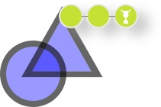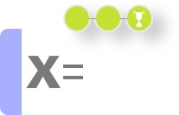# Check-as-you-go GeoGebra Tutorials

## GeoGebra Graphing and Geometry Courses

Learn how to use the GeoGebra Geometry App in 15 minutes with one of the following self-learning courses created by our friends from Kikora.
 Geometry App Courses Geometry I﻿﻿﻿﻿﻿ Lines, triangles, rectangles, and squares Geometry II﻿﻿﻿﻿﻿ Polygons, special triangles, angles, area, and perimeter## GeoGebra Classic Courses

Learn how to use GeoGebra Classic in 15 minutes with one of the following self-learning courses created by our friends from Kikora.
 Geometry Courses Geometry I﻿﻿﻿ Lines, triangles, rectangles, and squares Geometry II﻿﻿﻿ Polygons, special triangles, angles, area, and perimeterFunctions & Graphing Courses Functions and Graphs I﻿﻿﻿ Point coordinates and linear functions Functions and Graphs II﻿﻿﻿ Sliders and functions, special points, intersecting equationsComputer Algebra System (CAS) Courses CAS I﻿﻿ Simple calculations, algebraic terms, solving equations CAS II﻿﻿ Inequalities, factorization, substituting, assigning values CAS III﻿﻿ Radians and degrees, logarithms, more CAS hints## More Tutorials

Want to learn even more? Have a look at our Geometry App Tutorials, Graphing Calculator Tutorials or Classic App Tutorials on our Tutorials page.